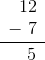ISEE Lower Level Quantitative : How to subtract

Example Questions

Example Question #357 : Isee Lower Level (Grades 5 6) Quantitative Reasoning

Subtract: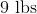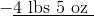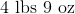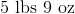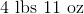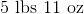Explanation:

One pound is equal to 16 inches, so: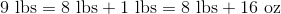In this equivalent problem, subtract pounds and ounces separately: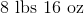Example Question #358 : Isee Lower Level (Grades 5 6) Quantitative Reasoning

Subtract: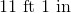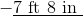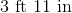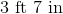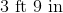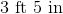Explanation:

One foot is equal to 12 inches, so: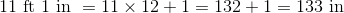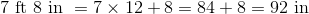We can now subtract inches: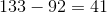inches, which can be converted to feet by dividing by 12: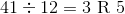, so

41 inches is equivalent to 3 feet, 5 inches.

Example Question #359 : Isee Lower Level (Grades 5 6) Quantitative Reasoning

Janet wins a bag of 60 marbles and wants to give some to Julie so that each girl will have the same number of marbles. Julie already has 40 marbles; Janet already has 30.  How many of the 60 marbles will Janet keep?Explanation:

After Janet wins the 60 marbles, the girls together own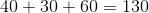marbles. Each should therefore own half this, or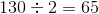marbles. Since Janet has 30 marbles already, she will keep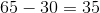marbles.

Example Question #360 : Isee Lower Level (Grades 5 6) Quantitative Reasoning

The time is now 8:08 PM. What time was it three hours and seventeen minutes ago?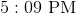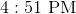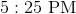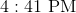Explanation:

You can subtract three hours and seventeen minutes from 8:08 PM as follows: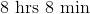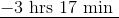However, since 17 minutes is greater than 8 minutes, the time can be rewritten as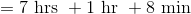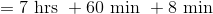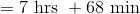Now subtract: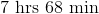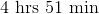That is, 4:51 PM.

Example Question #11 : How To Subtract

If a is even and b is odd, which of the following statements MUST ALWAYS be true about the value of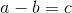?

c is negative

c is even

c is positive

c is odd

c is odd

Explanation:

Whenever an odd number is added or subtracted to or from an even number, the result MUST be odd. Therefore, the correct answer is "c is odd."

While subtracting an odd number from an even number CAN result in a positive or negative number, it will NOT always have that result.

Example Question #12 : How To Subtract

If an angle is equal to 145 degrees, how many more degrees is it than a right angle?Explanation:

If an angle is equal to 145 degrees, it is 55 degrees more than a right angle. This is because there are 90 degrees in a right angle, and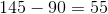. Thus, 55 is the correct answer.

Example Question #13 : How To Subtract

A roundtrip plane ticket from Los Angeles to Las Vegas costs $220. However, a one way ticket to Las Vegas costs$120 and a one way return flight costs $95 dollars. Which is travel method is cheaper? Possible Answers: Buying the roundtrip ticket because it is$5 cheaper.

Buying the roundtrip ticket because it is $10 cheaper. Buying the one way tickets because it is$5 cheaper.

Buying the one way tickets because it is $10 cheaper. Correct answer: Buying the one way tickets because it is$5 cheaper.

Explanation:

If a one way ticket to Las Vegas costs $120 and a one way return flight costs$95 dollars, the total cost is $115. If a roundtrip plane ticket from Los Angeles to Las Vegas costs$220, the one way tickets are \$5 cheaper.

Example Question #14 : How To Subtract

What is the value of the expression below?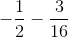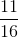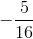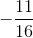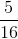Explanation:

First find a common denominator: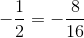The expression above can then be converted: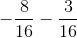Thus,is the correct answer.

Example Question #15 : How To Subtract

Martha baked a batch of 24 cookies and gave one fourth to her sister. How many cookies does she have left?Explanation:

If Martha baked a batch of 24 cookies and gave one fourth to her sister, she gave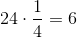cookies to her sister.

Given that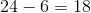, the number of remaining cookies is 18.

Example Question #3 : Use Addition And Subtraction Within 100 To Solve Word Problems Involving Lengths: Ccss.Math.Content.2.Md.B.5

The bowl isinches long and the plate isinches long. How much longer is the plate than the bowl?inchesinchesinchesinchesinchesinches